• https://jingyan.baidu.com/article/90808022a562b8fd90c80f64.html 转载于:https://www.cnblogs.com/DixinFan/p/9571557.html

https://jingyan.baidu.com/article/90808022a562b8fd90c80f64.html

转载于:https://www.cnblogs.com/DixinFan/p/9571557.html
展开全文• 今天给大家讲讲芯片数据分析中常见的一些图的作用，让大家伙儿知道它们在BB些啥。箱式图(Box plot)基因芯片的原始数据是需要进行标准化处理的，主要目的是消除由于实验技术(如荧光标记效率、扫描参数的设置、空间...今天给大家讲讲芯片数据分析中常见的一些图的作用，让大家伙儿知道它们在BB些啥。
箱式图(Box plot)
基因芯片的原始数据是需要进行标准化处理的，主要目的是消除由于实验技术(如荧光标记效率、扫描参数的设置、空间位置的差异等)所导致的基因表达量的变化，让各个样本和平行实验的数据处于相同的水平，使得基因表达数据真实地反映测量样品的生物学差异。
箱式图反映的是标准化前后的基因表达数据情况，标准化后，整体数据的中位数会处于同一水平线上(见下方右图)，这表明标准化的结果很好。常用的芯片间数据标准化方法有Quantile Normalization和Global Normalization。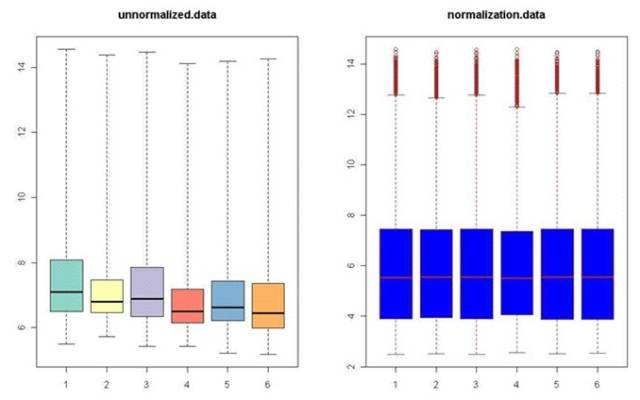散点图(Scatter plot)
芯片数据的散点图常用于评估两组数据总体分布集中趋势，是由芯片分析的原始数据经过标准化处理，转化为log2的对数后，在一个二维直角坐标系平面中绘制而成的。如果集中趋势不好，则表明芯片数据处理的不好(一般是标准化，归一化做的不好)。散点图中每个点代表一个探针信号，X轴Y轴数值分别对应该探针信号在不同样本中的强弱(下图X轴是Ctrl组，Y轴是Exp组)，图中的绿线是Fold change的阈值线(一般是±2)，即绿线之外的点在信号强弱(基因表达量)上是具有显著差异的。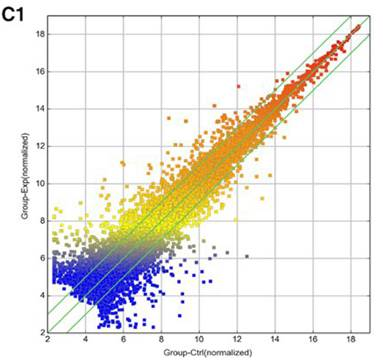聚类分析(Hierarchical cluster)图
聚类分析是为了寻找数据之间的相似性进行分类。基因芯片数据分析中比较常用的是分层聚类，它是利用一系列计算，首先找到关系最近(基因表达行为具有相关性等)的两群合并，再找关系相近两群再合并，直到所有的群合并到一个组中。用挑选的差异基因的表达情况来计算样本之间的相关性，对差异基因进行聚类分析可以全面地直观地展示样品之间的关系及差异情况(见下图)。一般来说，同一类样本能通过聚类出现在同一个簇(cluster)中(如果同一类样本，比如实验组3个样本，不能被聚类，则说明芯片分析的结果不好)，聚在同一个簇的基因可能具有类似的生物学功能。下图就是比较常见的聚类分析的热图(Heat map)。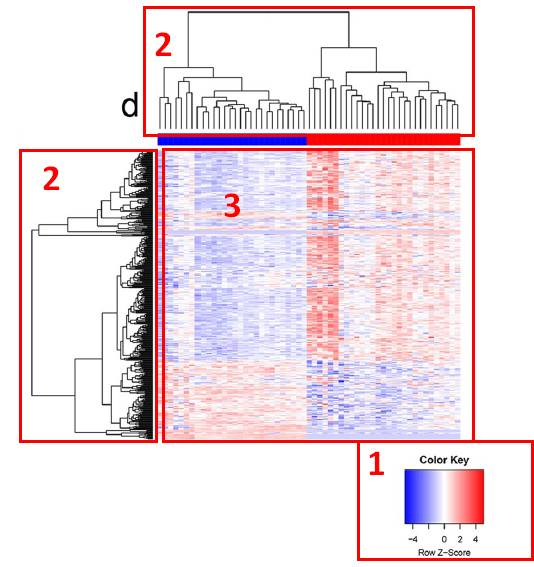1、色表，表示由蓝到红对应到基因表达量的变化，蓝色表示低表达，红色表示高表达；
2、树状图，X轴方向是样本的聚类，Y轴方向是基因的聚类；
3、每个色块代表一个基因的表达量，X轴对应所属样本，Y轴对应基因名称。
火山图(Volcano plot)
火山图就是长得像火山喷发的图(本宫真的没在瞎BB)。火山图在一张图中显示了两个重要的指标，Fold change和P-value，可以非常直观且合理地筛选出在两样本间发生差异表达的基因。比如下图，X轴是log(Fold change),Y轴为-log(P-value),设置FC和P-value的阈值筛选差异基因，那些红点表示的就是差异基因。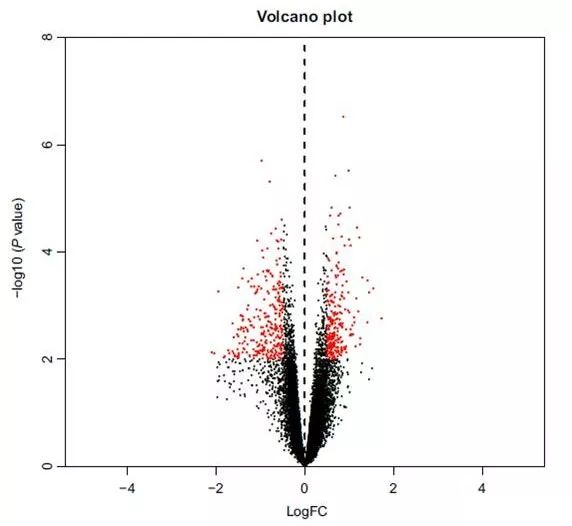GO图
在上次的文章(有关生物信息学你必须要知道的)中提到基因本体论(Gene ontology, GO)是对基因功能的注释，它由许多个词条构成，而这些词条是有层次的，它们具有从属关系，所以这些词条构成了一个有向无环图。GO的最顶层是细胞组分(Cellular Component, CC)，分子功能(Molecular Function, MF)和生物学过程(Biological Process, BP)这三个词条。下图就是一个GO富集分析的结果。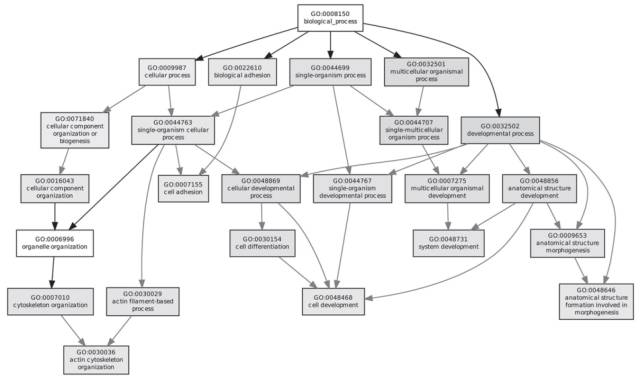维恩图(Venn diagrams)
维恩图用于显示元素集合重叠区域，举个应用的例子吧，芯片分析筛选出的差异表达基因是一个集合，数据库中查询的疾病相关基因是一个集合，我们把这两个集合取一个交集，这个交集中的基因就是我们可能会感兴趣的基因。下图中，作者定义了4个集合，4个集合均取交集的结果为0，所以作者只能退而求其次，选3个集合的交集。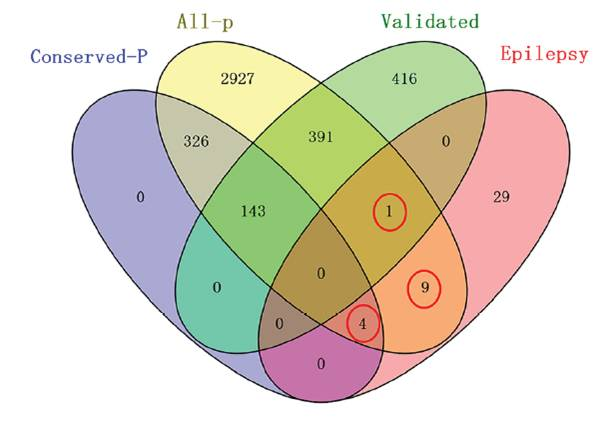文中插图的来源文献：
1、Exploring functions of long noncoding RNAs across multiple cancers through co-expression network
2、Microarray profiling analysis of long non-coding RNAs expression in tendinopathy: identification for potential biomarkers and mechanisms
3、Identification of differentially expressed genes and small molecule drugs for the treatment of tendinopathy using microarray analysis
4、
最后，再给大家推荐两篇文章练练手：
1、Circulating microRNA-150-5p as a novel biomarker for advanced heart failure: A genome-wide prospective study
2、Genome-wide analysis of long noncoding RNA (lncRNA) expression in colorectal cancer tissues from patients with liver metastasis
没有下载权限的童鞋可以去SCI-HUB下载(http://www.sci-hub.io/)。展开全文• T检验（Binary） T检验是一种适合小样本的统计分析方法，通过比较不同数据的均值，研究两组数据是否存在差异。 我们参考《python科学计算第二版》： ...

↑↑↑关注后"星标"Datawhale
每日干货 & 每月组队学习，不错过
Datawhale干货
作者：刘洋，中科院大学，Datawhale成员
一个模型中，很重要的技巧就是要确定训练集与测试集特征是否同分布，这也是机器学习的一个很重要的假设，但很多时候我们默认这个道理，却很难有方法来保证数据同分布。
T检验（Binary）
T检验是一种适合小样本的统计分析方法，通过比较不同数据的均值，研究两组数据是否存在差异。
我们参考《python科学计算第二版》：
单样本t检验
单样本t检验是样本均值与总体均值的比较问题。其中总体服从正态分布，从正态总体中抽样得到n个个体组成抽样样本，计算抽样样本均值和标准差，判断总体均值与抽样样本均值是否相同。
from scipy.stats import ttest_1samp
import numpy as np

print("Null Hypothesis:μ=μ0=30，α=0.05")
ages = [25,36,15,40,28,31,32,30,29,28,27,33,35]
t = (np.mean(ages)-30)/(np.std(ages,ddof=1)/np.sqrt(len(ages)))

ttest,pval = ttest_1samp(ages,30)
print(t,ttest)
if pval < 0.05:
print("Reject the Null Hypothesis.")
else:
print("Accept the Null Hypothesis.")
配对样本t检验
配对样本主要是同一实验前后效果的比较，或者同一样品用两种方法检验结果的比较。可以把配对样本的差作为变量，差值的总体均数为0，服从正态分布。
from scipy.stats import ttest_rel
s1 = [620.16,866.50,641.22,812.91,738.96,899.38,760.78,694.95,749.92,793.94]
s2 = [958.47,838.42,788.90,815.20,783.17,910.92,758.49,870.80,826.26,805.48]
print("Null Hypothesis:mean(s1)=mean(s2)，α=0.05")
ttest,pval = ttest_rel(s1,s2)
if pval < 0.05:
print("Reject the Null Hypothesis.")
else:
print("Accept the Null Hypothesis.")
独立样本t检验
对于第三个问题独立样本t检验，比较两个样本所代表的两个总体均值是否存在显著差异。除了要求样本来自正态分布，还要求两个样本的总体方差相等“方差齐性”。
from scipy.stats import ttest_ind,norm,f
import numpy as np
def ftest(s1,s2):
'''F检验样本总体方差是否相等'''
print("Null Hypothesis:var(s1)=var(s2)，α=0.05")
F = np.var(s1)/np.var(s2)
v1 = len(s1) - 1
v2 = len(s2) - 1
p_val = 1 - 2*abs(0.5-f.cdf(F,v1,v2))
print(p_val)
if p_val < 0.05:
print("Reject the Null Hypothesis.")
equal_var=False
else:
print("Accept the Null Hypothesis.")
equal_var=True
return equal_var

def ttest_ind_fun(s1,s2):
'''t检验独立样本所代表的两个总体均值是否存在差异'''
equal_var = ftest(s1,s2)
print("Null Hypothesis:mean(s1)=mean(s2)，α=0.05")
ttest,pval = ttest_ind(s1,s2,equal_var=equal_var)
if pval < 0.05:
print("Reject the Null Hypothesis.")
else:
print("Accept the Null Hypothesis.")
return pval

np.random.seed(42)
s1 = norm.rvs(loc=1,scale=1.0,size=20)
s2 = norm.rvs(loc=1.5,scale=0.5,size=20)
s3 = norm.rvs(loc=1.5,scale=0.5,size=25)

ttest_ind_fun(s1,s2)
ttest_ind_fun(s2,s3)
KS检验（Numerical）
KS检验是一种统计检验方法，其通过比较两样本的频率分布、或者一个样本的频率分布与特定理论分布（如正态分布）之间的差异大小来推论两个分布是否来自同一分布。
KS检验与t-检验之类的其他方法不同是KS检验不需要知道数据的分布情况，可以算是一种非参数检验方法。当然这样方便的代价就是当检验的数据分布符合特定的分布事，KS检验的灵敏度没有相应的检验来的高。在样本量比较小的时候，KS检验最为非参数检验在分析两组数据之间是否不同时相当常用。
PS：t-检验的假设是检验的数据满足正态分布，否则对于小样本不满足正态分布的数据用t-检验就会造成较大的偏差，虽然对于大样本不满足正态分布的数据而言t-检验还是相当精确有效的手段。
判断是否符合正态分布
KS函数说明文档：https://docs.scipy.org/doc/scipy/reference/generated/scipy.stats.kstest.html
import numpy as np
import pandas as pd
from scipy import stats

data = [87,77,92,68,80,78,84,77,81,80,80,77,92,86,
76,80,81,75,77,72,81,72,84,86,80,68,77,87,
76,77,78,92,75,80,78]
# 样本数据，35位健康男性在未进食之前的血糖浓度

df = pd.DataFrame(data, columns =['value'])
e = df['value'].mean()  # 计算均值
std = df['value'].std()  # 计算标准差
stats.kstest(df['value'], 'norm', (e, std))
# .kstest方法：KS检验，参数分别是：待检验的数据，检验方法（这里设置成norm正态分布），均值与标准差
# 结果返回两个值：statistic → D值，pvalue → P值
# p值大于0.05，为正态分布

#KstestResult(statistic=0.1590180704824098, pvalue=0.3066297258358026)
# p值大于0.05，不拒绝原假设，因此上面的数据服从正态分布。
#且一般情况下， stats.kstest(df[‘value’], ‘norm’, (u, std))一条语句就得到p值的结果。

#from scipy import stats
#stats.kstest(rvs, cdf, args=(),…)
#其中rvs可以是数组、生成数组的函数或者scipy.stats里面理论分布的名字
#cdf可以与rvs一致。若rvs和cdf同是数组，则是比较两数组的分布是否一致；一个是数组，另一个是理论分布的名字，则是看样本是否否和理论分布
#args是一个元组，当rvs或者cds是理论分布时，这个参数用来存储理论分布的参数，如正态分布的mean和std。
KL Divergence
KL 散度是一种衡量两个概率分布的匹配程度的指标，两个分布差异越大，KL散度越大。注意如果要查看测试集特征是否与训练集相同，P代表训练集，Q代表测试集，这个公式对于P和Q并不是对称的。
计算公式为:
对于离散分布
对于连续分布
import numpy as np
import scipy.stats

# 随机生成两个离散型分布
x = [np.random.randint(1, 11) for i in range(10)]
print(x)
print(np.sum(x))
px = x / np.sum(x)
print(px)
y = [np.random.randint(1, 11) for i in range(10)]
print(y)
print(np.sum(y))
py = y / np.sum(y)
print(py)

# 利用scipy API进行计算
# scipy计算函数可以处理非归一化情况，因此这里使用
# scipy.stats.entropy(x, y)或scipy.stats.entropy(px, py)均可
KL = scipy.stats.entropy(x, y)
print(KL)

# 实现
KL = 0.0
for i in range(10):
KL += px[i] * np.log(px[i] / py[i])
# print(str(px[i]) + ' ' + str(py[i]) + ' ' + str(px[i] * np.log(px[i] / py[i])))

print(KL)
机器学习模型检测
用特征训练模型来分辨测试集与测试集，若模型效果好的话代表训练集和测试集存在较大差异，否则代表训练集和测试集分布比较相似。
具体做法是构建一个二分类模型，对训练集打上0，测试集打上1，然后shuffle一下进行训练，若分类效果好，代表训练集和测试集区分度很高，那么分布差异就较大。整理不易，点赞三连↓

展开全文python 机器学习 人工智能 深度学习
一、获取数据 我们设定两组数据量差不多都是30w的数据的dat文件，地址（string）为dataAPath，dataBPath。通过地址读取数据byte[] 代码如下： // 通过地址读取数据 public byte[] readFile(String fileAddress){ InputStream in = null; ByteArrayOutputStream baos = new ByteArrayOutputStream(); byte[] content = null; try { // 一次读多个字节 byte[] tempbytes = new byte; int byteread = 0; in = new FileInputStream(fileAddress); // 读入多个字节到字节数组中，byteread为一次读入的字节数 while ((byteread = in.read(tempbytes)) != -1) { baos.write(tempbytes, 0, byteread); } content = baos.toByteArray(); } catch (Exception e) { e.printStackTrace(); } finally { if (in != null) { try { in.close(); } catch (IOException e1) { } } } return content; } 二、数据处理 byte 转 double(因为java里面的byte[-127,128],转完double也是16进制)代码如下： public double[] byte2double(byte[] data){ double[] byte2double = new double[Ad_data_size]; for(int i = 0; i < Ad_data_size; i++){ if(data[i]<0){ byte2double[i]=256+ data[i]; }else{ byte2double[i] = data[i]; } } return byte2double; } 再 16进制转10进制代码如下： public double[] sixteen2ten(double[]data){ double[] byte2double = new double[length]; for (int i = 0; i < length; i++) { byte2double[i]=data[2*i]256+data[2i+1]; } return byte2double; } 归一化处理，不然出现的数据跳跃性比较大代码如下： public double[] unitary(double[] data) { double data_avg=0; //求出平均值 for (int i = 0; i < data.length; i++){ data_avg += data[i]/(data.length); } //归一处理 for (int i = 0; i < data.length; i++) data[i] = (data[i] - data_avg) / data_avg; return data; } 三、傅里叶相关函数处理 代码如下： public double[] Rxy(double[] y1, double[] y2) { int N = y1.length; double[] y1_2N = new double[2 * N]; double[] y2_2N = new double[2 * N]; for (int i = 0; i < N; i++) { y1_2N[i] = y1[i]; y2_2N[i] = y2[i]; } for (int i = N; i < 2 * N; i++) { y1_2N[i] = y2_2N[i] = 0; } RealToComplex rtc = new RealToComplex(); Complex[] y1_C = rtc.ToComplex(y1_2N); Complex[] y2_C = rtc.ToComplex(y2_2N); FFT fft = new FFT(); Complex[] y1_fft = fft.fft(y1_C, 2 * N); Complex[] y2_fft = fft.fft(y2_C, 2 * N); Complex[] y2_conj = new Complex[2 * N]; Complex[] Sxy = new Complex[2 * N]; for (int i = 0; i < 2 * N; i++) { y2_fft[i].Conjugate(); y2_conj[i] = y2_fft[i]; Sxy[i] = fft.multiplication(y1_fft[i],y2_conj[i]); } Complex[] Sxy_ifft = fft.ifft(Sxy, 2 * N); double[] Sxy_ifft_real = new double[2 * N]; for (int i = 0; i < 2 * N; i++) { Sxy_ifft_real[i] = Sxy_ifft[i].real; } double[] Rxy = fft.fftshift(Sxy_ifft_real); return Rxy; } 实数转虚数代码 public class RealToComplex { public Complex[] ToComplex(double[] inputData) { int DataLength = inputData.length; Complex[] outputData = new Complex[DataLength]; for (int i = 0; i < DataLength; i++) { outputData[i] = new Complex(inputData[i], 0); } return outputData; } } 虚数定义代码 public class Complex { public double real; public double imag; public Complex(double real, double imaginary) { this.real = real; this.imag = imaginary; } public void Conjugate(){ imag = -imag; } } 傅里叶变换代码 public Complex[] fft(Complex[] Data, int N) { int r = (int)(Math.log(N) / Math.log(2)); Complex[] butt1 = new Complex[N]; Complex[] butt2 = new Complex[N]; for (int i = 0;i < N;i++) butt1[i] = Data[i]; Complex[] w = new Complex[N / 2]; for (int k = 0; k < N / 2; k++) { double angle = -k * Math.PI * 2 / N; w[k] = new Complex(Math.cos(angle), Math.sin(angle)); } for (int i = 0; i < r; i++) { int m = 1 << i; int n = 1 << (r - i); for (int j = 0; j < m; j++) { int index = j * n; for (int k = 0; k < n / 2; k++) { butt2[index + k] = add(butt1[index + k],butt1[index + k + n / 2]); butt2[index + k + n / 2] = multiplication(subtraction(butt1[index + k], butt1[index + k + n / 2]) , w[k * m]); } } for (int j = 0;j < N;j++) butt1[j] = butt2[j]; } for (int j = 0; j < N; j++) { int rev = 0; int num = j; for (int i = 0; i < r; i++) { rev <<= 1; rev |= num & 1; num >>= 1; } butt2[rev] = butt1[j]; } return butt2; } 虚数乘法运算代码 public Complex multiplication(Complex sum1,Complex sum2){ Complex summ = new Complex(0,0); summ.real = sum1.real * sum2.real - sum1.imag * sum2.imag; summ.imag = sum1.real * sum2.imag + sum1.imag * sum2.real; return summ; } fft的逆运算代码 public Complex[] ifft(Complex[] Data, int N) { for (int i = 0; i < N; i++) { //取共轭 Data[i].Conjugate(); } Complex[] butt = new Complex[N]; butt = fft(Data, N); for (int i = 0; i < N; i++) { butt[i] = real_division(butt[i] ,N); } return butt; } 傅里叶数据转移 public double[] fftshift(double[] Data) { int dataLength = Data.length; int harfLength; double[] OutPut = new double[dataLength]; if (dataLength % 2 == 0) { harfLength = dataLength / 2; for (int k = 0; k < harfLength; k++) { OutPut[k] = Data[harfLength + k]; OutPut[k + harfLength] = Data[k]; } } else { harfLength = (dataLength + 1) / 2; OutPut[harfLength - 1] = Data; for (int k = 0; k < harfLength - 1; k++) { OutPut[k] = Data[harfLength + k]; OutPut[k + harfLength] = Data[k + 1]; } } return OutPut; } 数据结果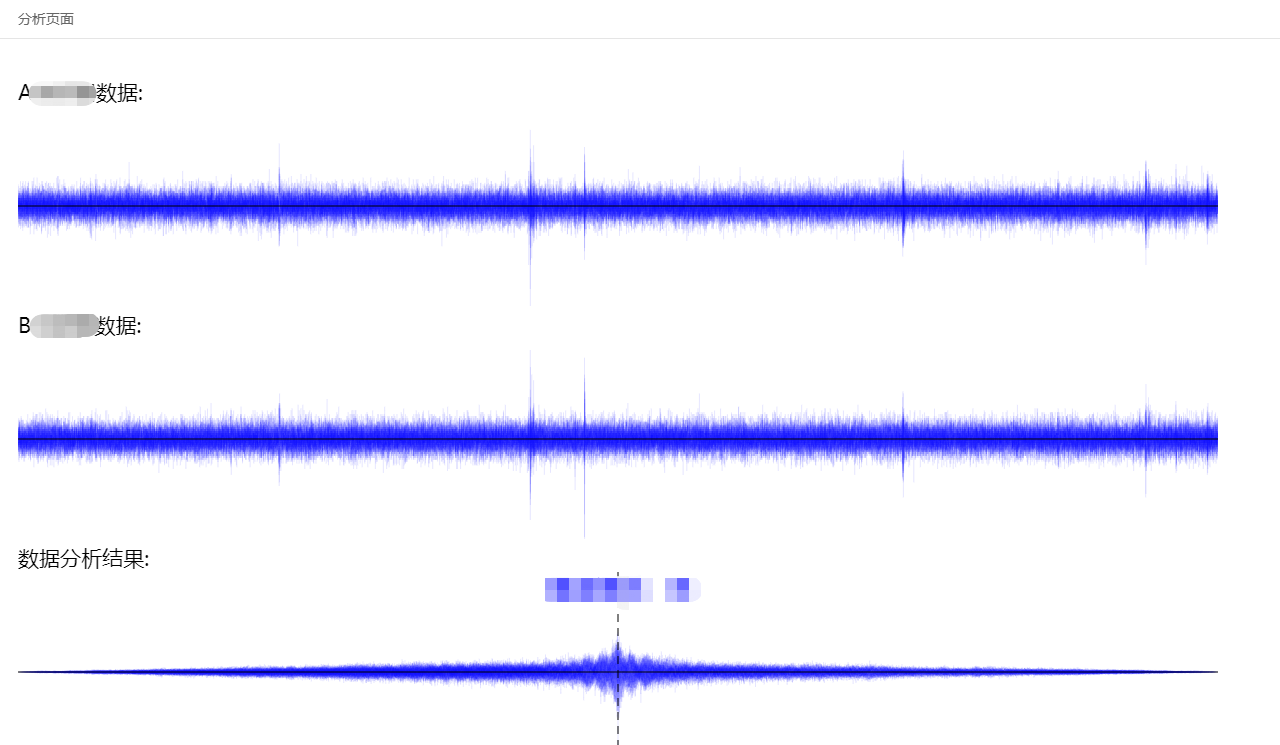展开全文傅里叶
• 叙述了传统的PCA方法在处理QAR数据相似性问题的不足，提出基于EROS的KPCA方法处理QAR数据之间的相似性问题。通过引入EROS方法而不需要对数据进行向量化，引入核矩阵对QAR数据进行主成分分析，可以有效降低数据的维数...
• r怎么对两组数据统计检验Business analytics and data science is a convergence of many fields of expertise. Professionals form multiple domains and educational backgrounds are joining the analytics ...python java 大数据 人工智能
• 怎么评价两组数据是否接近 接近组数据(组间) (Approaching group data (between-group)) A typical situation regarding solving an experimental question using a data-driven approach involves several groups ...python java 人工智能
• 这个问题比较有代表，对于两组或者两组以上的数据比较，其实 可以转化为诸多业务问题。例如，两组物流商，分别有两个月的运作时效数据，该如何对比、判断哪家运作物流商运作质量相对较优？再例如，有三个班次的...
• 通过两组统计数据计算而得的协方差可以评估这两组统计数据相似程度. 样本: A = [a1, a2, a3 ... an] B = [b1, b2, b3 ... bn] 求均值: ave_A = np.mean(A) ave_B = np.mean(B) 求离差: dev_A = [a1, a2, a3 .. ...
• ↑关注 + 星标~有趣的不像个技术号每晚九点，我们准时相约偶尔应金主爸爸要求改时间大家好，我是朱小五如何来展现的你的数据？是你有时不得不去思考的一个问题。不同的展示方法，其效果往往差异巨大。这里我将结合...
• Question 1：什么是ANOSIM分析？ ANOSIM分析（Analysis ...ANOSIM相似性分析是一种非参数检验，用来检验组间（两组或多组）差异是否显著大于组内差异，从而判断分组是否有意义。首先利用Bray-Curtis算法计算两两样品间
• 本文总结了数据分析和可视化中非常实用的几种图表，并以不同情景进行分组。01 为什么图表在科学出版物中很重要？复杂的数据有时可能难以用简洁的文字解释清楚，但却可以通过图表的形式予以直观...
• 今天，就让我们根据数据分析软件亿信ABI来解读一下各类统计图的使用场景以及优缺点。希望掌握这11种可视化图表，能够快速提升和巩固你的数据可视化展示，一起来看看吧！1、 柱状图适用场景：以柱子的高度用来比较两...
• ANOSIM 相似性分析 （非参数检验） 原理：首先利用 Bray-Curtis 算法计算两两样品间的距离，然后将所有距离从小到大进行排序。 目的：用来检验组间（两组或多组）的差异是否显著大于组内差异，从而判断分组是否...
• 所以 cosß=a2+c2−b22accos_ß=\frac {a^2+c^2-b^2}{2ac}cosß​=2aca2+c2−b2​ 任意两个坐标的余弦公式 回归正题，我们最终目的是为了求两组数据的相似度，那能不能把每组数据当作一个坐标呢？也可以说当作两个...算法 人工智能 大数据 java
• 序 时间序列是一类最常见的数据，目前时间序列分析重点研究的多是时间序列的预测。但针对有些问题，时间序列的形态比较也是一类重要的问题。例如：各种商品每日均价（或者股票的每日收盘价格）构成了时间序列，如何...
• 尝试使用seurat包进行两组间差异分析 使用的是seurat包自带的数据 #首先载入需要的包 library(Seurat) #安装seurat-data包 install.packages('devtools') library("devtools") devtools::install_github('satijalab...r语言 python
• 概述：简介、思路、发展...数据分析方法：描述统计、假设检验、信度分析、相关分析、方差分析、回归分析、聚类分析、判别分析、主成分分析、因子分析、时间序列分析等； 数据可视化：Excel、PowerBI、Tableau、Python；python 大数据
• 实验选取两组某烟草企业提供的烟叶光谱构建了光谱的相似性度量模型, 以相似性度量的准确率作为算法优劣的衡量标准。实验结果表明, GGLLE算法构建的相似性度量模型的准确率为93.3%, 明显优于主成分分析、栈式自编码器...
• 在上一篇文章数据挖掘：探索性数据分析(EDA)中，已经讨论了一些探索性分析的方法，但去敏数据的处理方式和一些多元统计的方法没有做介绍。本篇文章主要讲这两方面。 一、去敏数据的处理方式 去敏数据已经在之前有过...机器学习
• 相似性和相异性被许多数据挖掘技术所使用，如聚类、最近邻分类、异常检测等。两个对象之间的相似度是这两个对象相似程度的数值度量，通常相似度是非 负值，并常常在0（不相似）和1（完全相似）之间取值。两个对象...
• 这次课程主要讲述一个关于Kmeans聚类的数据分析案例，通过这个案例让同学们简单了解大数据分析的基本流程，以及使用Python实现相关的聚类分析。 主要内容包括： 1.Anaconda软件的安装过程及简单配置 2.聚类及...python 聚类 Kmeans
• ## Python 数据相关性分析

万次阅读 多人点赞 2018-04-25 15:09:49
会有一个这样的场景，有若干数据罗列在我们的面前，这组数据相互之间可能会存在一些联系，可能是此增彼涨，或者是负相关，也可能是没有关联，那么我们就需要一种能把这种关联定量的工具来对数据进行分析，从而给...python 相关性 pandas numpy
• 第5章 数据的描述性分析 通过前面两章的学习，我们知道，数据收集是取得统计数据的过程，数据预处理是将数据中的问题清理干净，那么接下来的步骤就是统计分析了。数据分析是通过统计方法研究数据的过程，所用的...R语言 机器学习
• 天津大学《数据分析数据挖掘》公开课–学习笔记 1.1 数据分析数据挖掘 数据分析是指采用适当的统计分析方法对收集到的数据进行分析、概括和总结，对数据进行恰当的描述，提取出有用的信息的过程。对决策进行辅助...数据库 python sql
• 在机器学习和数据挖掘中，我们经常需要知道个体间差异的大小，进而评价个体的相似性和类别。最常见的是数据分析中的相关分析数据挖掘中的分类和聚类算法，如 K 最近邻（KNN）和 K 均值（K-Means）等等。根据数据...机器学习
• 在机器学习和数据挖掘中，我们经常需要知道个体间差异的大小，进而评价个体的相似性和类别。最常见的是数据分析中的相关分析数据挖掘中的分类和聚类算法，如 K 最近邻（KNN）和 K 均值（K-Means）等等。根据数据...机器学习 曼哈顿距离 欧氏距离
• 点击上方蓝字关注我们对比分析数据分析中最常用的、最好用、最实用分析方法之一。没有对比就不能说明问题，这也是对比分析数据分析领域经久不衰的原因之一。对比分析是将两个或两个以上具有可比的...大数据 人工智能 python 机器学习
• 在机器学习和数据挖掘中，我们经常需要知道个体间差异的大小，进而评价个体的相似性和类别。最常见的是数据分析中的相关分析数据挖掘中的分类和聚类算法，如 K 最近邻（KNN）和 K 均值（K-Means）等等。根据数据...机器学习 公式 方法
• 在机器学习和数据挖掘中，我们经常需要知道个体间差异的大小，进而评价个体的相似性和类别。最常见的是数据分析中的相关分析数据挖掘中的分类和聚类算法，如 K 最近邻（KNN）和 K 均值（K-Means）等等。在做分类时...距离度量 机器学习...